# Anatomy of a Program

Many of the tutorials for Processing concentrate on what the language can do (change colors, draw shapes, create arrays of objects) and which function calls will let you accomplish these tasks. These are things you need to know in order to write a program in Processing.

There’s one piece of the puzzle that these tutorials don’t address: how do you analyze a problem and break it down into steps that the computer can do? In this tutorial, I’m going to show you what went through my head as I took on the task of writing functions to draw regular polygons and star-shaped figures in Processing. This is a good choice because the task is not too big to get your mind around, but it’s not an entirely trivial problem, either.

Remember that what you are seeing here is my particular thought process and programming style. There are many different approaches and styles. As you continue programming, you will find your own. You can also see other people’s programming stye (though not their thought process!) by looking at the source code for the programs at openProcessing.org.

### Drawing Regular Polygons

You wouldn’t think of building a house without a blueprint, and you shouldn’t think of writing a program without a plan of some sort. Since Processing is such a visual language, I always have to sketch out what I want as a result before I approach the keyboard. So that’s where I started.

### Step 1: Planning on paper

The first step was to draw a crude diagram to dredge up old memories of how regular polygons work. The hexagon was the first one I drew. As you can see from the little loop I drew inside the hexagon, the center angles of all the slices add up to a complete circle, or 360°, and the “radius” of the polygon is a line from the center to each vertex. The angle between each of the radius lines is thus 360° divided by the number of sides.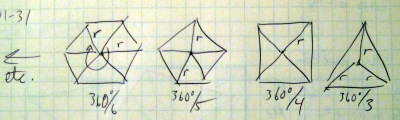I just needed to get an idea of what the task was, and a hand-drawn diagram did the job. I didn’t need to generate the diagram in a drawing program.

Programming Hint: When you do your planning, do it away from the computer. If you are sitting in front of the computer, the screen will whisper, “Look at me! Look at me!” and the keyboard will whisper “Type something! Type something!” Go to your kitchen table instead.

### Step 2: Some basic trigonometry

So, if you have a line of length r starting at (0,0) at an angle theta (θ), what are its coordinates in terms of x and y? If you know a little bit of trigonometry, the answer is that the endpoint of the line is at (r cos θ, r sin θ). If you don’t know trigonometry, take a look at this tutorial (a general and very light introduction to trigonometry), this tutorial (oriented towards Processing), and this example from chapter 13 of Learning Processing. In the following diagram, angles are drawn clockwise, which is how they are measured in Processing.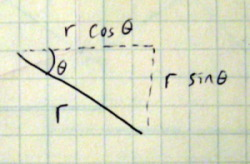### Step 3: Design Decisions

It looks like this is a job for a `for` loop that runs from 0 to n (the number of sides), calculating the points for each vertex and drawing lines connecting them. At each step, the angle at which we draw is increased by 360°/n.

The problem with drawing a group of lines is that they are just lines—you don’t get a true shape that you can fill, like `rect()` or `triangle()`. Luckily, Processing lets you create your own shapes with the `beginShape()`, `vertex()`, and `endShape()` functions. The first example on the reference page for `beginShape()` is the model to follow. So the next design decision is to make polygons as true shapes.

Since you probably want to draw many polygons during a program, it makes sense to have a `polygon()` function. What parameters does it need? Four come to mind: the number of sides, the center x and y coordinate, and the radius. Here’s the code. I wrote several different calls to `polygon()` in the `setup()` function. Although I calculated `angle` in degrees, sine and cosine measure angles in radians, so I had to use the `radians()` function to do a conversion.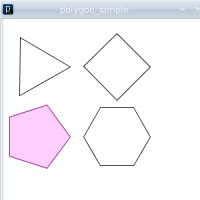```def setup():
size(300, 300)
background(255)
smooth()

noFill()
polygon(3, 50, 75, 50)
polygon(4, 170, 75, 50)

fill(255, 204, 255)
stroke(128, 0, 128)
polygon(5, 50, 180, 50)

noFill()
stroke(0)
polygon(6, 170, 180, 50)

def polygon(n, cx, cy, r):
angle = 360.0 / n

beginShape();
for i in xrange(n):
vertex(cx + r * cos(radians(angle * i)),
cy + r * sin(radians(angle * i)))
endShape(CLOSE)

```

### Two Steps Forward, One Step Back

The program works, so it’s time to see if there are things that could be added or changed. First, the triangle and pentagon seem somehow wrong; they are usually drawn pointing upwards instead of to the side. The reason they look odd is that the first vertex (at 0°) points to the right instead of straight up. It would be nice to have an extra parameter that gives the starting angle for the first vertex. (Another solution is to leave things as they are and let programmers use `rotate()` [see this tutorial], but I made the design decision to use an extra parameter.) Should the angle be given in degrees or in radians? The answer: radians, in order to be consistent with everything else that Processing does.

My next thought was that it would be nice to be able to specify a width and height for the polygon, much as you do with an `ellipse()` or `rectangle()`. I already knew what the formula would be, but I wanted to make a drawing to check it out. As a preliminary experiment, I tried drawing a pentagon into a square using a protractor and straightedge, and ended up with the awful drawing at the left. How come the sides weren’t equal length? I realized that I was trying to make the drawing fit my preconceptions, rather than making an accurate drawing and seeing where that led me. The drawing on the right was done much more carefully. After a little thinking, I realized that the pentagon wouldn’t fit the square exactly, because the angles weren’t multiples of 90 degrees. The regular polygon fits in a circle, not in a square!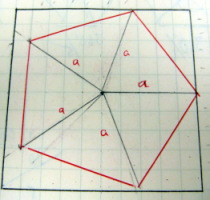Well, that was a dead end. That sort of thing happens in programming all the time, so I didn’t spend too much time worrying about it. It was time for another approach. Since I didn't have an accurate way of drawing ellipses, I had to think about the problem a different way. Presume you have a circle drawn on a square sheet of rubber, and you stretch it out so that it’s twice as wide but the same height. The vertical position of the points on the circle does not change, but the horizontal positions are now twice as far away from the center as they used to be. The same idea applies if you stretch the sheet vertically. The following crude drawings seemed to bear this out, so it was time to rewrite the `polygon()` function.```def setup():
size(300, 300)
background(255)
smooth()

noFill()
polygon(3, 50, 75, 100, 100, -PI / 2.0)   # -90 degrees
polygon(4, 170, 75, 50, 125, -PI / 4.0)   # -45 degrees

fill(255, 204, 255)
stroke(128, 0, 128)
polygon(5, 50, 200, 75, 50, -PI / 2.0)    # -90 degrees

noFill()
stroke(0)
polygon(6, 170, 200, 50, 100, 0)

stroke(128)
# draw enclosing ellipses to make sure we did it right
ellipse(50, 75, 100, 100)
ellipse(170, 75, 50, 125)
ellipse(50, 200, 75, 50)
ellipse(170, 200, 50, 100)

def polygon(n, cx, cy, w, h, startAngle):
angle = TWO_PI/ n

# The "radius" is one half the total width and height
w = w / 2.0
h = h / 2.0

beginShape()
for i in xrange(n):
vertex(cx + w * cos(startAngle + angle * i),
cy + h * sin(startAngle + angle * i))
endShape(CLOSE)

```

Since everything was in radians, I now described angles in terms of `PI` and `TWO_PI` (2π), since 2π radians equals 360°. In addition to the code in `setup()` to test the new features, I drew ellipses with the same center and width and height as the polygons to make sure that the vertices were within the proper area.

### Too Many Parameters

I now had a much more flexible function for drawing polygons, but it came at the expense of more parameters. It would be nice to be able to draw the ordinary case (equal width and height, start angle of zero) without having to specify all those parameters. The solution is to use default parameters. In Python, you can have certain parameters to a function act as defaults, meaning if the user does not define them our defaults will step in. This means that a function can be called with a different number of parameters depending on what the user would like to do! An example of this is Processing’s `stroke()` function, which allows you to call it with a single number for grayscale, three numbers for color, or four numbers for color with opacity.

Here is what we'll change the definition of our `polygon()` function to look like to adjust for default parameters. Notice how we've changed our fourth parameter `w` to `r`, this is (stylistically) to account for the fact that we can now represent width and height as r * 2.0. Within our function, we can now make a check to see if the user defined an `h` and `startAngle` and then adjust our parameters accordingly.

```def polygon(n, cx, cy, r, h = None, startAngle = None):
w = h = startAngle = 0 # Define these variables outside the scope of the if statement
if (h == None and startAngle == None): # User defined 4 parameters
# If not, adjust our parameters.
w = r * 2.0
h = r * 2.0
startAngle = 0
else: # User defined 6 parameters
w = r

```

And here is some code to test the overloaded function.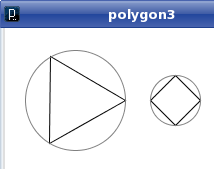```def setup():
size(300, 300)
background(255)
smooth()

noFill()
polygon(3, 70, 75, 50)   # use the defaults
polygon(4, 170, 75, 25)

stroke(128)
# draw enclosing ellipses to make sure we did it right
ellipse(70, 75, 100, 100)
ellipse(170, 75, 50, 50)

```

### Safe Computing

What happens if someone tries to draw a polygon with 2 sides, 1 side, or worse, 0 sides? The first two will generate a line and a point, but the third one will cause a division by zero error when trying to figure out the angle. And what would happen with negative numbers? Since polygons with fewer than three sides don’t make a lot of sense, I wrapped the body of the `polygon()` function inside of an `if` statement. Now, when someone specifies two or fewer sides, the function just won’t draw anything.

```def polygon(n, cx, cy, r, h = None, startAngle = None):
if (h == None and startAngle == None): # User defined 4 parameters
# If not, adjust our parameters.
w = r * 2.0
h = r * 2.0
startAngle = 0
else: # User defined 6 parameters
w = r

if (n > 2):
angle = TWO_PI/ n;
.
.
beginShape()
.
.
endShape(CLOSE)

```

### Drawing Stars

Now that I was satisfied with the `polygon()` function, it was time to move on to drawing stars. From some playing around with a crude sketch, I figured that it would be possible to draw a star by figuring out where all the diagonals of a polygon intersect.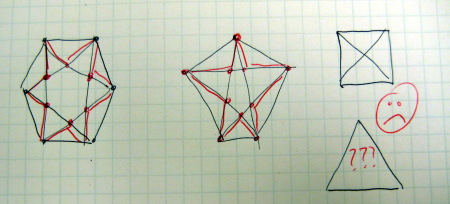I saw two problems here. First, finding the intersection point of two lines is a lot of calculation. Not particularly hard calculation, but a lot of it, and it gets tricky when you have vertical lines. Second, I couldn't have a four-sided or three-sided arrow shape; there weren’t enough diagonals.

Then, I had another idea. I can’t tell you where the idea came from or how I arrived at it; it just hit me. That’s a part of the process that I don’t think can be taught. Here was the idea: What if you had a polygon cut out of cookie dough and you sort of pushed in the sides to make a star shape? That is a method that works for squares and triangles.When you push in the sides, you push them in at the midpoint so that you get a nice symmetric cookie. From there, it wasn’t a big leap to figure out: “what if I had a shorter radius at half of every slice of the polygon?”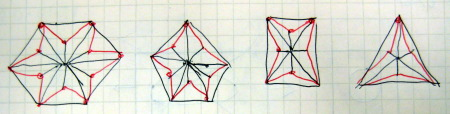This code would be fairly easy to write. I would need one extra parameter: the proportion of the small radius to the big radius. In the following code, an `if` statement controls whether to use the short radius or the long radius. I also put in an overloaded version that draws a star with equal width and height and a start angle of zero. For the test, I made the short radius one half the long radius. Notice how on the simpler calls, the user specifies `proportion = x` within the call.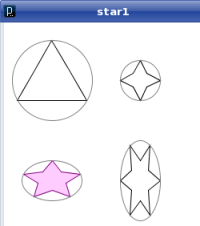```def setup():
size(300, 300)
background(255)
smooth()

noFill()
star(3, 60, 75, 100, 100, -PI / 2.0, 0.50)   # -90 degrees
star(4, 170, 75, 25, proportion = 0.50)   # use simpler call

fill(255, 204, 255)
stroke(128, 0, 128)
star(5, 60, 200, 75, 50, -PI / 2.0, 0.50)    # -90 degrees

noFill()
stroke(0)
star(6, 170, 200, 50, 100, 0, 0.50)
stroke(128)

# draw enclosing ellipses to make sure we did it right
ellipse(60, 75, 100, 100)
ellipse(170, 75, 50, 50)
ellipse(60, 200, 75, 50)
ellipse(170, 200, 50, 100)

def star(n, cx, cy, r, h = None, startAngle = None, proportion = 1.0):
if (h == None and startAngle == None): # User defined 4 parameters
# If not, adjust our parameters.
w = r * 2.0
h = r * 2.0
startAngle = 0
else: # User defined 6 parameters
w = r

if (n > 2):
angle = TWO_PI/ (2*n)   # twice as many sides
dw = 0  # draw width
dh = 0  # draw height

w = w / 2.0
h = h / 2.0

beginShape()
for i in xrange(2 * n):
print dw, w, h, dh
dw = w
dh = h
if (i % 2 == 1): # for odd vertices, use short radius
dw = w * proportion
dh = h * proportion
vertex(cx + dw * cos(startAngle + angle * i),
cy + dh * sin(startAngle + angle * i))
endShape(CLOSE)

```

### What Went Wrong?

When I ran this program, I just freaked out. Everything looked great, except for the three-sided star. How come I didn’t get a star from it? The code sure looks correct, so I decided to see what would happen if I drew the diagram by hand. I measured the angles with my protractor, and I drew the long radius lines with a length of two inches in black and the short radius lines with a length of one inch in red. Sure enough, it just so happens that the endpoints of the short radius lines are right on the lines of the main triangle. The program is drawing a star, with the sides pushed in by zero.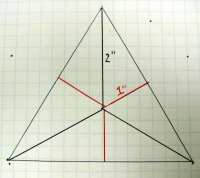While wondering why this happened, I remembered that the cosine of the angle between the lines, 60° (π/3) comes out to 0.5, and I strongly suspected that this had something to do with it. To test my hypothesis, I changed the square to use a proportion of cosine of 45° (π/4), the pentagon to cosine of 36° (π/5), and the hexagon to cosine of 30° (π/6). Sure enough, they all came out with no push-in.

So, if you are drawing a star with n sides and you set the proportion for the short radius to long radius to the cos(π/n), you get a non-star star! I still can’t write a mathematical proof of it, but it is an interesting result. I don’t consider that side trip of writing the test program to be wasted time; I did learn something new and mildly interesting, and it may turn out to be useful in the future.

Programming Challenge: What happens if you set the proportion to something greater than the “non-star” ratio? Try it and find out.

Of course, the way to get a three-sided star is to set the proportion to an amount less than 0.5, which I did in the following setup code, with much better results. I also changed the proportions for the other stars just to see what they would look like.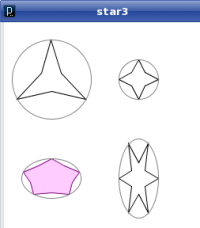```def setup():
size(300, 300)
background(255)
smooth()

noFill()
star(3, 60, 75, 100, 100, -PI / 2.0, 0.3)   # -90 degrees
star(4, 170, 75, 25, proportion = 0.5)   # use simpler call

fill(255, 204, 255)
stroke(128, 0, 128)
star(5, 60, 200, 75, 50, -PI / 2.0, 0.75)   # -90 degrees

noFill()
stroke(0)
star(6, 170, 200, 50, 100, 0, 0.4)
stroke(128)

# draw enclosing ellipses to make sure we did it right
ellipse(60, 75, 100, 100)
ellipse(170, 75, 50, 50)
ellipse(60, 200, 75, 50)
ellipse(170, 200, 50, 100)

```

### Using the Functions

Finally, in order to use the functions in something other than a test, I decided to write a program that would randomly generate polygons and stars. The window is 300 by 300, and the stars or polygons have a radius of 24 dots, so I have six rows and five columns (the extra dot is for spacing). Remember how I said that knowing the proportions that would create a star “may turn out to be useful in the future”? Well, they aren’t just useful for this program—they’re vital. When I generate a star, I need to make sure it really has a star shape, so I have to keep the proportion of short to long radius less than the cosine of π divided by the number of sides.

Here is the code for `setup()` and `draw()`; I am not including the code for the `polygon()` and `star()` functions here; it is the same as in the examples.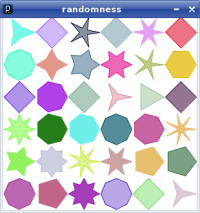```def setup():
size(300, 300)
background(255)
frameRate(6)
smooth()
rectMode(CENTER)

def draw():
# choose a random stroke color
r = int(random(0, 255))
g = int(random(0, 255))
b = int(random(0, 255))
# and fill opacity
opacity = int(random(100, 255))
nSides = int(random(3, 9))

# determine the center x and y coordinates
cx = 25 + 50 * int(random(0, 6))
cy = 25 + 50 * int(random(0, 6))

# if a random number (0 or 1) is 0, draw a polygon;
# otherwise, draw a star
isPolygon = int(random(2)) == 0

# for stars, you need the proportion of short to long radius
# proportion

stroke(255)   # erase any previous drawing in this area
fill(255)
rect(cx, cy, 50, 50)

stroke(r, g, b)
fill(r, g, b, opacity)
if (isPolygon):
polygon(nSides, cx, cy, 24)
else:
proportion = random(0.2, 0.8) * cos(PI / nSides)
star(nSides, cx, cy, 24, proportion)

```

### Polygons and Stars as Objects

Now that I have working functions for polygons and stars, it might be useful to make a `Polygon` and `Star` class so that I can treat them as objects. The method I would use is much the same; I would start with simple test cases, build up the classes step by step, and finally use them in a full-blown program. Here is a tutorial about objects in Processing.

### Summing Up

This tutorial has shown you the things you never see in books. In a book, all the diagrams are picture perfect. You see a sample program, and it just works, and it produces gorgeous results. To be fair, the authors can’t show you their thought process; otherwise, their books would be ten times as large. In fact, I did not include all the versions where a misplaced parenthesis or a forgotten call to `radians()` made my sketch explode into a mass of incomprehensible lines. But all of us, the big name authors, the people who write these tutorials, and the beginning programmers, go through this same tawdry process of design, trial and error, and development. I wanted you to see that process at least once, because we are all in this together.